# Nexxushost Forums

• August 22, 2019, 02:34:45 AM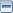• Welcome, Guest
Please login or register.

Login with username, password and session length
Pages: 1 

###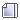AuthorTopic: are you guys interested in any math stuff?  (Read 6371 times)

#### AsinineVulpine

• Full Member
•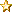• Posts: 170##### Re: are you guys interested in any math stuff?
« Reply #15 on: November 04, 2014, 02:45:01 PM »

(sorry; slowly writing a post on derivatives, then integration, then the quadratic formula, then differential equations. busy week & weekend)
(<3)Logged

#### Dr. Javi

• Full Member
•• Posts: 167##### Re: are you guys interested in any math stuff?
« Reply #16 on: November 08, 2014, 09:55:35 AM »

Next:
1) Derivatives (Rusti)
2) Integration (Rusti)
3) the Quadratic Formula (Bane & Jade)
4) Differential Equations (Rusti)

Derivatives
Here's where calculus starts!

If a car is driving 60 miles-an-hour, then the rate of change of its position at any given instant is 60 miles-an-hour. On the other hand, if a car isn't moving at all, then the rate of change of its position is zero, since its position is... well, not changing. Mathematically, we call this rate of change a derivative. It is, in short, the answer to the question: "At this point in time, what is the rate of change of a particular value?"

Since everything changes in some way—the idea of making a derivative mathematically rigorous is hugely valuable. It does not always suffice to estimate (guestimate isn't a word i hate you) a value's rate of change. Building something like the derivative is not a trivial thing, though—so we start with something simpler and build up! To be explicit, here's the game plan: We're going to take a fairly simple function and consider its average rate of change over a period of time. From that, we'll derive a general equation for a derivative:

Consider a series of values, say, the position of a car over time: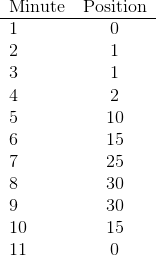So the car starts off at 0 mph. for the first minute, stays at 1 mph for a couple of minutes, creeps up to 2 mph (annoying driver), etc. We can, if we'd like, call this f(x), where x is a time-value. For example, f(10) = 15. Now, say I were to ask "What is the rate of change of the car's position from 6 minutes to 7 minutes?" To answer this question, one might follow these steps:

1) Look at the above chart (looking is a necessity).
2) Take the car's position at 6 minutes.
3) Take the car's position again at 7 minutes.
4) Subtract these two values from one another, getting a total change of position between 6 and 7 minutes.
5) Divide this value by the amount of time that passed between 6 and 7 minutes—the difference between 6 and 7.

Mathematically, the above steps describe: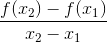Where, in our case, x1 = 6 and x2 = 7. Remember, (f(x) is the car's position at time x). The value that results from performing this is, then, the amount that the car's position changed in the span between 6 and 7 minutes. It is the rate of change of the car's position between these two points in time. Similarly, if we want the average rate of change of the car's position between any two points, we follow a very similar method.

What if we want the average rate of change between minute 3 and minute 8? Here, we perform an identical action: First, we take the car's position at minute 3 and subtract it from its position at minute 8 (30 - 1 = 29). Then, we divide this number by the total time that passed between minutes 3 and 8—5 minutes. We thus have: 29 ➗ 5 = 5.8. It's kind of a shitty number, but it does give us the average rate of change of the car's position (per minute) between those two times!

We can check this: At minute 3, the car's position is 1. Let's add 5.8 to its position for every minute until we get to minute 8. Thus, at minute 4—its position is 6.8. 5 is 12.6, 6 is 18.4, 7 is 24.2, and 8 is 30, which is what we wanted. On average, the car moved 5.8 miles per minute, from minute 3 to minute 8.

Note, though... this is not exact. What if, at minute 3, the car sped to position 30 in one minute—then stayed still until minute 8. We'd still get the same average rate of change. The rate of change would still be 5.8, because the rate doesn't depend on what happens in the middle. This average, while sometimes useful, does not accurately describe the motion of the car.

What do?

Well... how can we get a more accurate idea of what the car is doing in the middle?

We'd have to consider the average of a smaller chunk of time. Instead of considering the chunk from 3 minutes to 8 minutes, we can take from 3 minutes to 5 minutes, or 3 minutes to 4 minutes. If we had the data, we could even take from 3 minutes to 3.01 minutes! This'd give us much more accurate description of what's going on between these two times. The smaller we go, the more accurate our "average" rate of change is.... which brings us much closer to what we want—an instantaneous rate of change.

What if our chunk of time was infinitely small? Say our initial value is x, and let's say ε is an infinitely small number.  ε is infinitely smaller than the smallest number you can think of. It's infinitely smaller than all numbers. It's little. So, let's say that the time we want is between x and x + ε; an infinitely tiny chunk of time. We can apply the exact same action we did above, where our second time is x2 = x + ε.

Thus, we have: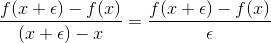This is the instantaneous rate of change. The immediate rate of change of your function at any given point. It's difficult to apply this to the above car-example, since the data we have for the car is in fairly large intervals—and we just don't have access to what's going on at minute 3.25, much less minute 3 + ε. But what if we did? What if the car's position is given as f(x) = x2? Then we could plug this right in and get a function for the instantaneous rate of change of x2 at any x.

This is exactly, mathematically, the derivative of a function.

Ok, good. We've found what the derivative is—and we have a formula for it. If we plug various functions in, we can find their derivatives... but it's often obnoxious to work with that definition. Try it with f(x) = x2; one will eventually get the correct answer w/ some use of algebra, but it's annoying.

Is there a way to just take the derivative of a general function?

Yes. Yes, there is. I won't prove it (I could in another post if people are interested—it just takes a bit), but I will explain how to do it!

It turns out that the derivative of x2 is 2x. Similarly, the derivative of x3 is 3x2, and the derivative of x4 is 4x3. Notice a pattern?

The derivative of xn is nxn-1. Basically, multiply your function by the exponent and subtract one. This holds for a large range of functions. Say... (x + 3)3. The derivative of this is 3(x + 3)2. From this, we can derive a bunch of different rules that help in taking the derivative of more complicated functions, such as (x2 + 3x)4, where there are multiple exponents to work with.

Would anyone be interested in these other rules/methods/etc.?

Was this helpful at all to anyone? Is there too much description, not enough description? Too technical? Not technical enough? Are there things you don't understand or need some clarification? Is there not enough motivation for the derivative—does it seem like I just jump into the math?

Was this too basic? Is this what you were looking for when you mentioned the derivative (SPECIFICALLY YOU, RUSTI. YOU SPECIFICALLY)?

Everyone feel free to ask as many/whatever questions you want; I like them. Criticize anything and everything without bound!

« Last Edit: November 09, 2014, 09:49:32 AM by Dr. Javi »Logged
you can clearly see that budapestian bitch has gotta' be soaked in the knickers all fanning herself off

#### AsinineVulpine

• Full Member
•• Posts: 170##### Re: are you guys interested in any math stuff?
« Reply #17 on: November 09, 2014, 07:25:24 AM »

aw man that is awesome to see.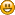sadly my brain apparently has failed to process mathematics yet again. :<  I can feel it wanting to fully comprehend, I can feel that "ah-HAH!" just somewhere very close by but I can't seem to grab it and it's slightly frustrating. I don't think that's your fault at all, Javi, because that's an awesome, fun explaination, but I think my brain is just refusing to process mathematics of that level at the moment and I can't for the life of me figure out why. I might just be tired as fuck. Carry on with the integration and differential equations though because I still wanna see them and they might help someone else too.Logged

#### Dr. Javi

• Full Member
•• Posts: 167##### Re: are you guys interested in any math stuff?
« Reply #18 on: November 09, 2014, 09:41:48 AM »

A couple things that might help: That "ah-HAH!" moment doesn't always accompany understanding (in fact, a lot of times it doesn't accompany at all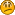); often times you have to work with it, and it's got to stew in your mind for a while.

Ask me questions. If you don't have any questions ready, then think of it and find some! If you don't have any immediate questions, it means you don't know exactly what you're not understandingwhich is often related to the feeling of being on the edge of understanding; "I know I can understand this, but there's something about it I'm not getting." Find that something (figuring out questions to ask is really helpful with this).

Even if it's "What algebra steps did you take to get to [this] step?" Ask any question you possibly can.

(Also, I could even make some exercise-type problems if you want to practice.)Logged
you can clearly see that budapestian bitch has gotta' be soaked in the knickers all fanning herself off
Pages: 1